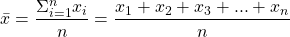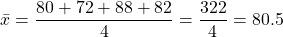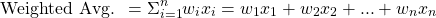# Working With Weighted Averages

in StemSocial2 months ago

Hi there. In this post, I would like to cover weighted averages. The usual average or arithmetic mean assumes that every value is weighted the same. What if you wanted a balanced score where each value had a different weight? You would need a weighted average.

One common example would be test and assignment scores for a school course. Not all tests, assignments and exams are weighted equally. A test is typically weighted more than a quiz and a test is weighed less than a final exam.

Weighted averages are also used in finance but the calculations are a little bit different that the one presented in this post. That would be another topic.

The math text is rendered in LaTeX with the use of images done with QuickLatex.com.Pixabay Image Source

## Topics

• Review Of The Usual Average
• Computing Weighted Averages With Examples

## Review Of The Usual Average

The word average refers to the one average where the numbers in a list or data set are added together first. This sum or total is then divided by the number of values to obtain a single value that represents a centre point for the list of numbers.

Here is the formula for the usual arithmetic mean or average:Example

The average of the math test scores 80, 72, 88 and 82 would be computed as:This student's average from the four math scores is 80.5. The usual average assume that each of the four tests are weighted equally. That is each math test is worth 25% each.

What if each test is weighted differently? A lot of school courses do not weigh things the same with their tests, assignments, projects, presentations and exams. We need the weighted average to account for different weights.Pixabay Image Source

## Computing Weighted Averages

Even though weighted averages require more calculations in the form of addition and multiplication, they are more flexible and adaptable to more real life situations. Weighted averages are very helpful for scenarios such as school courses with different weightings on assignments, tests and exams and for finance.

General Formula

Given the weights `w1, w2, ... wn` and the scores/values `x1, x2, to xn`, the general formula for computing the weighted average is this:Note that `n` is the number of values. In addition the weights all add up to 1 to represent 100%. The usage of this formula is better seen through examples.

Example One

Melanie has these scores from her three math tests and a final exam with their corresponding weights. This is displayed in a table format below.

EvaluationWeightScore
Test #120%76
Test #220%81
Test #330%80
Final Exam30%86

What is Melanie's final grade?

The weights can be converted into decimals for helping with calculations. As the weights for the evaluations are not all the same, you cannot use the usual average where you add all the scores and divide by four. You have to multiply each of the weights with their respective scores. After getting each weighted score, add all of them to obtain Melanie's final grade. I present the answer in a table format.

EvaluationWeightScoreWeighted Score
Test #120%760.2 x 76 = 15.2
Test #220%810.2 x 81 = 16.2
Test #330%800.3 x 80 = 24
Final Exam30%860.3 x 86 = 25.8
Course Grade100%N/A81.2

Example Two

There are courses in university where the final exam has a heavy weight. I have heard rumors that some math courses at the University of Waterloo have final exams that have a weight of 70% of the final grade. Kinda crazy to me as that kind of course rewards good test takers or something.

Suppose that Michael is taking a math course where the final exam does have a 70% weight on the final grade. The only other evaluation is a 30% midterm test. Here are the results from Michael's math course.

EvaluationWeightScore
Midterm Test30%92
Final Exam70%74

Determine Michael's final grade for this math course. What can you learn from this example?

The weighted score for Michael's midterm test is `0.3 x 92 = 27.6`. Michael's weighted score from the final exam is `0.7 x 74 = 51.8`.

Adding the two weighted scores of 27.6 and 51.8 gives the course grade of 79.4 for Michael. This is a B+ or maybe even an A if bumped up to 80. There are cases when professors get graded on a curve.

A lower final exam score than the midterm test not only reduces your course grade but it reduces it by quite a bit. Final exams that are worth that much should be taken seriously and it also adds pressure on test taking abilities.Pixabay Image Source

Example Three - What Score Do I Need?

This scenario does happen quite a bit amongst students. I am not sure about other universities but my undergraduate university had a thing where there was a final date that you can drop out of the course without failure. You would stay pay the fees for the days that you have attended class prior to the dropout. Dropping out of the course after this final date would result in a fail for the course.

This final date would typically occur after a midterm test has been graded. Students would know how they are doing in the course and can
decide to continue or not.

Jill has an abstract real analysis math course with three assignments, a midterm test and a final exam. She is faced with a decision of dropping out of this tough math course or sticking it out after the final date for course cancellations without failure. Here are Jill's results thus far.

EvaluationWeightScore
Assignment #110%61
Assignment #210%67
Midterm Test30%45
Assignment #310%Not Taken Yet
Final Exam40%Not Taken Yet

Jill just got back her results from the midterm test. After the final cancellation date, she has one more assignment and a final exam.

**What is Jill's course grade so far? **

What does Jill need on the final exam to pass the course (50%) assuming that she gets a 50% on the third assignment?

If Jill miraculously gets perfect on the third assignment and the final exam, what is the maximum grade she can get?

Answer

The weighted score from assignment one is `0.1 x 61 = 6.1`.

From assignment two, the weighted score is `0.1 x 67 = 6.7`.

The weighted score from the midterm test is `0.3 x 45 = 13.5`.

At the point, Jill has received 26.3 points out of 50. Jill has a course grade of **52.6% ** after the midterm test. Without looking at the second question, you can say that Jill would need to pass the third assignment and the final exam to pass the course.

Answer To Question Two

If Jill scores a 50% on the third assignment, she earns 5 points from that assignment. This would bring her points amount to 31.3 out of 55 which is 56.9%.

To pass the course Jill needs enough points to bring her points to 50. She needs 18.7 points from the final exam's 40 in order to pass. Percentage wise Jill needs a 46.75% on the final exam to pass the course.

Answer To Question Three

Scoring one hundred percent on the third assignment and the final exam will net Jill 10 points on top of the 26.3 points she had. She would have 76.3 points out of 100 which is 76.3% or a B grade. This is unlikely.Pixabay Image Source

Thank you for reading.

Sort:Thanks for your contribution to the STEMsocial community. Feel free to join us on discord to get to know the rest of us!

Please consider supporting our funding proposal, approving our witness (@stem.witness) or delegating to the @stemsocial account (for some ROI).

Please consider using the STEMsocial app app and including @stemsocial as a beneficiary to get a stronger support.